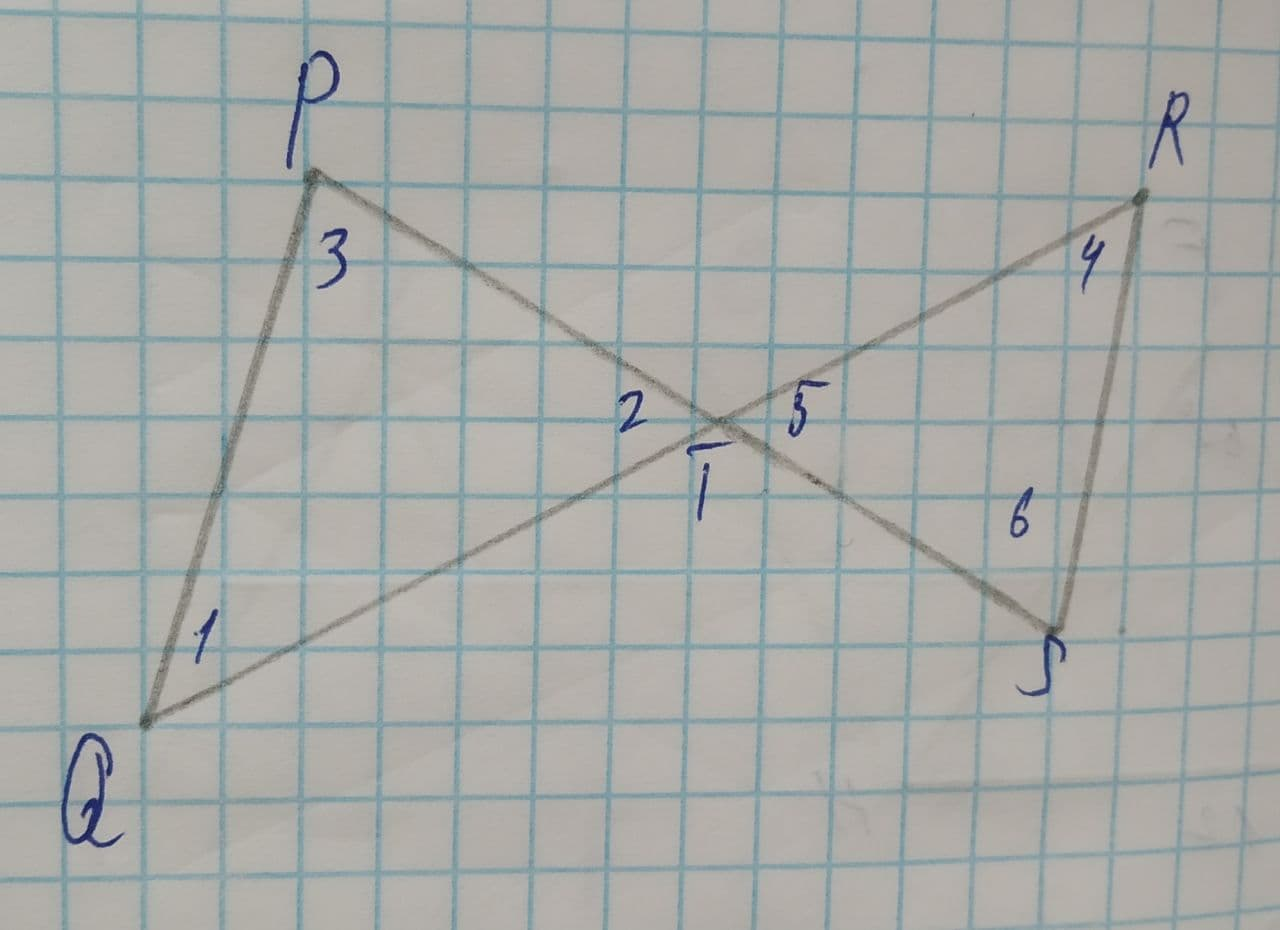Question# To explain: what can be said about the lines \overline{PQ}\ and\ \overline{RS}

Congruence
ANSWEREDTo explain: what can be said about the lines $$\displaystyle\overline{{{P}{Q}}}\ {\quad\text{and}\quad}\ \overline{{{R}{S}}}$$, it is given that $$\displaystyle\angle{1}\stackrel{\sim}{=}\angle{2}\ {\quad\text{and}\quad}\ \angle{4}\stackrel{\sim}{=}\angle{5}$$.
Given:
The diagram:2021-07-29

Concept Used:
Theorem 2-3: Vertically opposite angles are congruent.
Transitive property of congruence: if $$\displaystyle{a}\stackrel{\sim}{=}{b}\ {\quad\text{and}\quad}\ {b}\stackrel{\sim}{=}{c}$$, then $$\displaystyle{a}\stackrel{\sim}{=}{c}$$.
Theorem 3-5: If two lines are cut by a transversal and alternate interior angles are congruent, then the lines are parallel.
Consider the given figure:In this figure, by theorem 2-3,
$$\displaystyle\angle{2}\stackrel{\sim}{=}\angle{5}$$ (Vertically opposite angles)
Also, it is given that,
$$\displaystyle\angle{1}\stackrel{\sim}{=}\angle{2}\ {\quad\text{and}\quad}\ \angle{4}\stackrel{\sim}{=}\angle{5}$$
Now applying the transitive property of congruence, it can be said that
$$\displaystyle\angle{1}\stackrel{\sim}{=}\angle{4}$$
From the above figure, it can be observed that the angles $$\displaystyle\angle{1}\ {\quad\text{and}\quad}\ \angle{4}$$ are the alternate interior angles which are formed when the lines $$\displaystyle\overline{{{P}{Q}}}\ {\quad\text{and}\quad}\ \overline{{{R}{S}}}$$ are cut by the transversal $$\displaystyle\overline{{{Q}{R}}}$$.
And since $$\displaystyle\angle{1}\stackrel{\sim}{=}\angle{4}$$, so by the theorem 3-5, it can be said that the lines $$\displaystyle\overline{{{P}{Q}}}\ {\quad\text{and}\quad}\ \overline{{{R}{S}}}$$ are parallel.